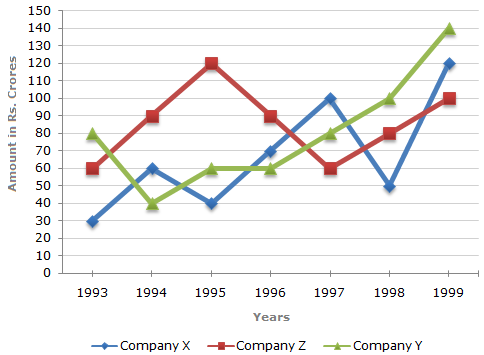# Data Interpretation - Line Charts - Discussion

Study the following line graph and answer the questions.

Exports from Three Companies Over the Years (in Rs. crore)3.

In which year was the difference between the exports from Companies X and Y the minimum?

 [A]. 1994 [B]. 1995 [C]. 1996 [D]. 1997

Explanation:

The difference between the exports from the Companies X and Y during the various years are:

In 1993 = Rs. (80 - 30) crores = Rs. 50 crores.

In 1994 = Rs. (60 - 40) crores = Rs. 20 crores.

In 1995 = Rs. (60 - 40) crores = Rs. 20 crores.

In 1996 = Rs. (70 - 60) crores = Rs. 10 crores.

In 1997 = Rs. (100 - 80) crores = Rs. 20 crores.

In 1998 = Rs. (100 - 50) crores = Rs. 50 crores.

In 1999 = Rs. (140 - 120) crores = Rs. 20 crores.

Clearly, the difference is minimum in the year 1996.

 Vijay said: (Aug 28, 2011) I am not able to understand it.

 Saheli Das said: (Sep 22, 2011) Just observe the graph and you can see that the difference between the exports from the Companies X and Y during 1996 is the least that is =(70-60)=10 , whereas for the other years it is 20 or more.

 Zakaria said: (Apr 29, 2012) No need to calculation, just see the difference of point between x and y line. the minimum difference between two point of these two line at 1996. so, ans is 1996.

 Rekharekha said: (Nov 19, 2014) Don't calculate all years just look at the years given in the option.

 Sachin said: (Sep 19, 2016) Sir, we just subtract x - y or we subtract small number from bigger?

 Malvee Singla said: (Oct 7, 2017) In this question, are we to only judge the magnitude or do we need to see the sign of the difference as well because in some cases X-Y is negative and in some it is positive?

 Manav said: (Jun 26, 2019) Yes, you are correct, Agree @Zakaria.